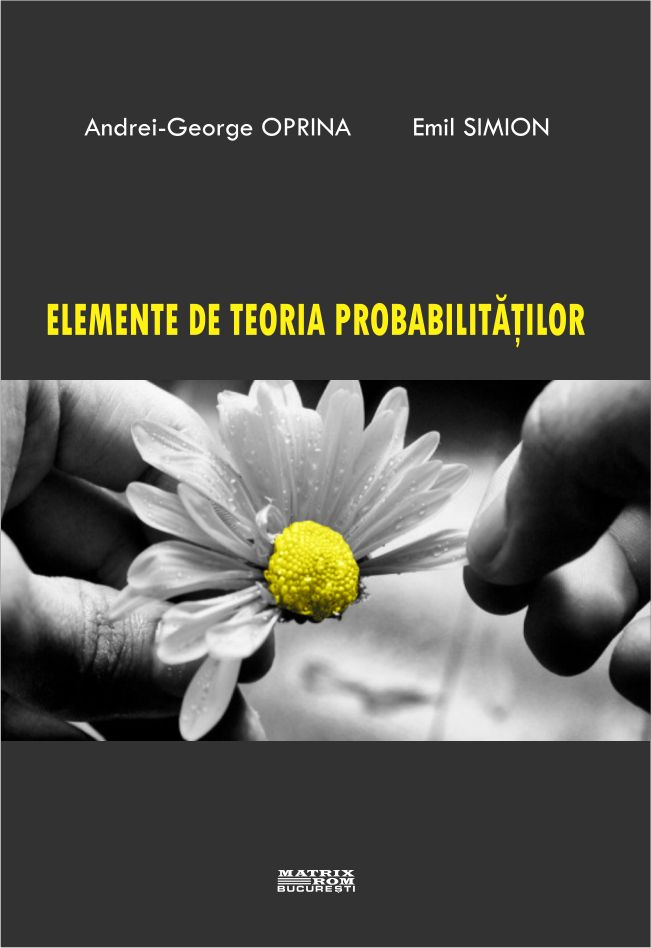English# Elements of probability theory

Universitatea Politehnica Bucuresti
ISBN: 978-606-25-0317-8
Limba: Romana
Suport: Hartie
36,00 lei
The use of probabilistic models is a widely used technique in all fields that require automatic data processing. The classical notion of probability refers to the study of randomness and uncertainty. In situations where the number of occurrences of the possible outcomes of an event can be quantified numerically, classical theory defines probability as the ratio of the number of favorable cases to the number of total cases. However, there are situations in which this is not possible, which is why an analytical or axiomatic mathematical apparatus is necessary.